# Previous Years GATE Questions on Heat Exchanger

## Solved GATE Questions on Heat Exchanger

### Question 1. For the same inlet and outlet temperatures of hot and cold fluids, the Log Mean Temperature Difference (LMTD) is

(A) Greater for parallel flow heat exchanger than for counter flow heat exchanger.

(B) Greater for counter flow heat exchanger than for parallel flow heat exchanger.

(C) Same for both parallel and counter flow heat exchangers.

(D) Depends on the properties of fluid.

GATE-ME-2002

Hint 1. (Ans B)

### Question 2. In a counter flow heat exchanger, for the hot fluid the heat capacity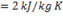, mass flow rate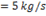, inlet temperature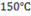, outlet temperature is 100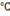. For the cold fluid, heat capacity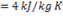, mass flow rate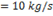, inlet temperature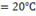. Neglecting heat transfer to the surroundings, the outlet temperature of the cold fluid in 0. is

(A) 7.5

(B) 32.5

(C) 45.5

(D) 70.0

GATE-ME-2003

Hint 2. (Ans B)

For hot fluid

Heat capacity,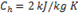Mass flow rate,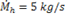Inlet temperature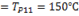Outlet temperature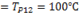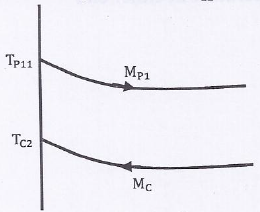Similarly for cold fluid

Heat capacity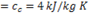Mass flow rate,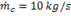Inlet temperature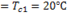Outlet temperature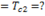Since Heat gain=Heat loss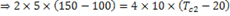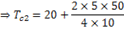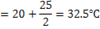### Question 3. Hot oil is cooled from 80to 50.in an oil cooler which uses air as the coolant. The air temperature rises from 30. To 40. The designer uses a LMTD value of 26. The type of heat exchanger is

(A) Parallel flow

(B) Double pipe

(C) Counter flow

(D) Cross flow

GATE-ME-2005

Hint 3. (Ans D)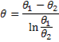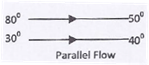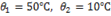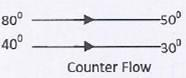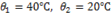As given value is between these two, so cross flow is the best option.

### Question 4. In a counter flow heat exchanger, hot fluid enters at 60. And cold fluid leaves at 30. Mass flow rate of the fluid is 1 kg/s and that of the cold fluid is 5 kJ/kg K. The Log Mean Temperature Difference (LMTD) for the heat exchanger inis

(A) 15

(B) 30

(C) 35

(D) 45

GATE-ME-2007

Hint 4. (Ans B)

Heat capacity of hot fluid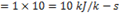Heat capacity of cold fluid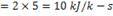Since heat capacity is same, so LMTD is difference of temperature at either end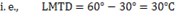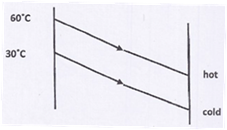### Question 5. The logarithmic mean temperature difference (LMTD) of a counter flow heat exchanger is 20. The cold fluid enters at 20and the hot fluid enters at 100. Mass flow rate of the cold fluid is twice that of hot fluid. Specific heat at constant pressure of the hot fluid is twice that of hot fluid. Specific heat at constant pressure of the hot fluid is twice that of cold fluid. The exit temperature of the cold fluid

(A) is 40(B) is 60(C) is 80(D) cannot be determined

GATE-ME-2008

Hint 5. (Ans C)

### Question 6. In a parallel flow heat exchanger operating under steady state, the heat capacity rates (product of specific heat at constant pressure and mass flow rate) of the hot and cold fluid are equal. The hot fluid, flowing at 1 kg/s with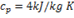, enters the heat exchanger at 102, while the cold fluid has an inlet temperature of 15. The overall heat transfer coefficient for the heat exchanger is estimated to be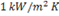and the corresponding heat transfer surface area is 5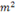.Neglect heat transfer between the heat exchanger and the ambient. The heat exchanger is characterized by the following relation: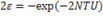### The exit temperature (in) for the cold fluid is

(A) 45

(B) 55

(C) 65

(D) 75

GATE-ME-2009

Hint 6. Ans (B)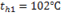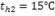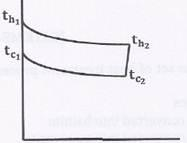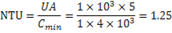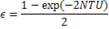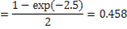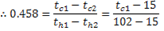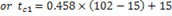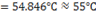### Question 7. In a condenser of a power plant, the steam condenser is at a temperature of 60. The cooling water enters at 30and leaves at 45. The logarithmic mean temperature difference (LMTD) of the condenser is

(A) 16.2(B) 21.6(C) 30(D) 37.5GATE-ME-2011

Hint 7.(Ans B)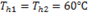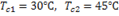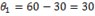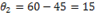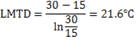1. (B)     2. (B)     3. (D)     4. (B)     5. (C)     6. (B)     7. (B)

### 2 Responses to “Previous Years GATE Questions on Heat Exchanger”

1.#### sai

Knowledgeable

2.#### fikremariam muluken

i like so much